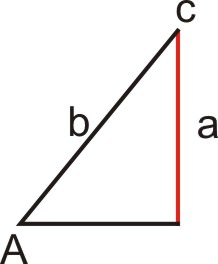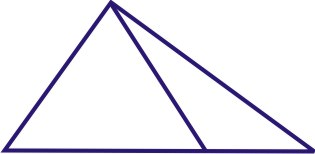# 4.1.5: Side-Side-Angle- The Ambiguous Case

$$\newcommand{\vecs}{\overset { \rightharpoonup} {\mathbf{#1}} }$$ $$\newcommand{\vecd}{\overset{-\!-\!\rightharpoonup}{\vphantom{a}\smash {#1}}}$$$$\newcommand{\id}{\mathrm{id}}$$ $$\newcommand{\Span}{\mathrm{span}}$$ $$\newcommand{\kernel}{\mathrm{null}\,}$$ $$\newcommand{\range}{\mathrm{range}\,}$$ $$\newcommand{\RealPart}{\mathrm{Re}}$$ $$\newcommand{\ImaginaryPart}{\mathrm{Im}}$$ $$\newcommand{\Argument}{\mathrm{Arg}}$$ $$\newcommand{\norm}{\| #1 \|}$$ $$\newcommand{\inner}{\langle #1, #2 \rangle}$$ $$\newcommand{\Span}{\mathrm{span}}$$ $$\newcommand{\id}{\mathrm{id}}$$ $$\newcommand{\Span}{\mathrm{span}}$$ $$\newcommand{\kernel}{\mathrm{null}\,}$$ $$\newcommand{\range}{\mathrm{range}\,}$$ $$\newcommand{\RealPart}{\mathrm{Re}}$$ $$\newcommand{\ImaginaryPart}{\mathrm{Im}}$$ $$\newcommand{\Argument}{\mathrm{Arg}}$$ $$\newcommand{\norm}{\| #1 \|}$$ $$\newcommand{\inner}{\langle #1, #2 \rangle}$$ $$\newcommand{\Span}{\mathrm{span}}$$$$\newcommand{\AA}{\unicode[.8,0]{x212B}}$$

Evaluate triangles given two sides and one angle not between them

## Possible Triangles with Side-Side-Angle

Your team has just won the flag in a flag football tournament at your school. As a reward, you get to take home the flag and keep it until the next game, when the other team will try to win it back. The flag looks like this:Figure $$\PageIndex{1}$$

It makes an isosceles triangle. You start to wonder how many different possible triangles there are for different lengths of sides. For example, if you make an oblique triangle that has a given angle greater than ninety degrees, how many ways are there to do this? Can you determine how many different possible triangles there are if the triangle is an isosceles triangle?

### SSA Triangles

In Geometry, you learned that two sides and a non-included angle do not necessarily define a unique triangle.

Consider the following cases given $$a$$, $$b$$ and $$\angle A$$:

Case 1: No triangle exists ($$a<b$$)Figure $$\PageIndex{2}$$

In this case $$a<b$$ and side $$a$$ is too short to reach the base of the triangle. Since no triangle exists, there is no solution.

Case 2: One triangle exists ($$a<b$$)Figure $$\PageIndex{3}$$

In this case, $$a<b$$ and side $$a$$ is perpendicular to the base of the triangle. Since this situation yields exactly one triangle, there is exactly one solution.

Case 3: Two triangles exist ($$a<b$$)Figure $$\PageIndex{4}$$

In this case, $$a<b$$ and side $$a$$ meets the base at exactly two points. Since two triangles exist, there are two solutions.

Case 4: One triangle exists ($$a=b$$)Figure $$\PageIndex{5}$$

In this case $$a=b$$ and side $$a$$ meets the base at exactly one point. Since there is exactly one triangle, there is one solution.

Case 5: One triangle exists ($$a>b$$)Figure $$\PageIndex{6}$$

In this case, $$a>b$$ and side $$a$$ meets the base at exactly one point. Since there is exactly one triangle, there is one solution.

Case 3 is referred to as the Ambiguous Case because there are two possible triangles and two possible solutions. One way to check to see how many possible solutions (if any) a triangle will have is to compare sides $$a$$ and $$b$$. If you are faced with the first situation, where $$a<b$$, we can still tell how many solutions there will be by using a and $$b\sin A$$.

If: Then:
a. $$a<b$$ No solution, one solution, two solutions
i. $$a<b\sin A$$ No solution
ii. $$a=b\sin A$$ One solution
iii. $$a>b\sin A$$ Two solutions
b. $$a=b$$ One solution
c. $$a>b$$ One solution

#### Identifying Triangles

For the following problems, determine if the sides and angles given determine no, one or two triangles.

1. The set contains an angle, its opposite side and the side between them.

$$a=5$$, $$b=8$$, $$A=62.19^{\circ}$$

$$5<8$$, $$8 \sin62.19^{\circ} =7.076$$. So $$5<7.076$$, which means there is no solution.

2. The set contains an angle, its opposite side and the side between them.

$$c=14$$, $$b=10$$, $$B=15.45^{\circ}$$

Even though $$a$$, $$b$$ and $$\angle A$$ is not used in this example, follow the same pattern from the table by multiplying the non-opposite side (of the angle) by the angle.

$$10<14$$, $$14\sin15.45^{\circ} =3.73$$. So $$10>3.73$$, which means there are two solutions.

3. The set contains an angle, its opposite side and the side between them.

$$d=16$$, $$g=11$$, $$D=44.94^{\circ}$$

Even though $$a$$, $$b$$ and $$\angle A$$ is not used in this example, follow the same pattern from the table by multiplying the non-opposite side (of the angle) by the angle.

$$16>11$$, there is one solution.

##### Example $$\PageIndex{1}$$

Earlier, you were given a problem about a triangle.

Solution

As you now know, when two sides of a triangle with an included side are known, and the lengths of the two sides are equal, there is one possible solution. Since an isosceles triangle meets these criteria, there is only one possible solution.

##### Example $$\PageIndex{2}$$

Determine how many solutions there would be for a triangle based on the given information and by calculating $$b \sin A$$ and comparing it with a. Sketch an approximate diagram for each problem in the box labeled “diagram.”

Solution

$$A=32.5^{\circ}$$, $$a=26$$, $$b=37$$

$$A=32.5^{\circ}$$, $$a=26$$, $$b=37$$

$$26>19.9$$Figure $$\PageIndex{7}$$

2 solutions

##### Example $$\PageIndex{3}$$

Determine how many solutions there would be for a triangle based on the given information and by calculating $$b \sin A$$ and comparing it with a. Sketch an approximate diagram for each problem in the box labeled “diagram.”

Solution

$$A=42.3^{\circ}$$, $$a=16$$, $$b=26$$

$$A=42.3^{\circ}$$, $$a=16$$, $$b=26$$

$$16<17.5$$Figure $$\PageIndex{8}$$

0 solutions

##### Example $$\PageIndex{1}$$

Determine how many solutions there would be for a triangle based on the given information and by calculating $$b \sin A$$ and comparing it with a. Sketch an approximate diagram for each problem in the box labeled “diagram.”

Solution

$$A=47.8^{\circ}$$, $$a=13.48$$, $$b=18.2$$

$$A=47.8^{\circ}$$, $$a=13.48$$, $$b=18.2$$

$$13.48=13.48$$Figure $$\PageIndex{9}$$

1 solution

### Review

Determine if the sides and angle given determine no, one or two triangles. The set contains an angle, its opposite side and another side of the triangle.

1. $$a=6$$, $$b=6$$, $$A=45^{\circ}$$
2. $$a=4$$, $$b=7$$, $$A=115^{\circ}$$
3. $$a=5$$, $$b=2$$, $$A=68^{\circ}$$
4. $$a=7$$, $$b=6$$, $$A=34^{\circ}$$
5. $$a=5$$, $$b=3$$, $$A=89^{\circ}$$
6. $$a=4$$, $$b=4$$, $$A=123^{\circ}$$
7. $$a=6$$, $$b=8$$, $$A=57^{\circ}$$
8. $$a=4$$, $$b=9$$, $$A=24^{\circ}$$
9. $$a=12$$, $$b=11$$, $$A=42^{\circ}$$
10. $$a=15$$, $$b=17$$, $$A=96^{\circ}$$
11. $$a=9$$, $$b=10$$, $$A=22^{\circ}$$
12. In $$\Delta ABC$$, $$a=4$$, $$b=5$$, and $$m\angle A=32^{\circ}$$. Find the possible value(s) of $$c$$.
13. In $$\Delta DEF$$, $$d=7$$, $$e=5$$, and $$m\angle D=67^{\circ}$$. Find the possible value(s) of $$f$$.
14. In $$\Delta KQD$$, $$m\angle K=20^{\circ}$$, $$k=24$$, and $$d=31$$. Find $$m\angle D$$.
15. In $$\Delta MRS$$, $$m\angle M=70^{\circ}$$, $$m=44$$, and $$r=25$$. Find $$m\angle R$$.

To see the Review answers, open this PDF file and look for section 5.9.

## Vocabulary

Term Definition
Side Side Angle Triangle A side side angle triangle is a triangle where the length of two sides and one of the angles that is not between the two sides are known quantities.